# Configuring Advanced Settings

Create custom calculation in the Advanced section of the KPI page.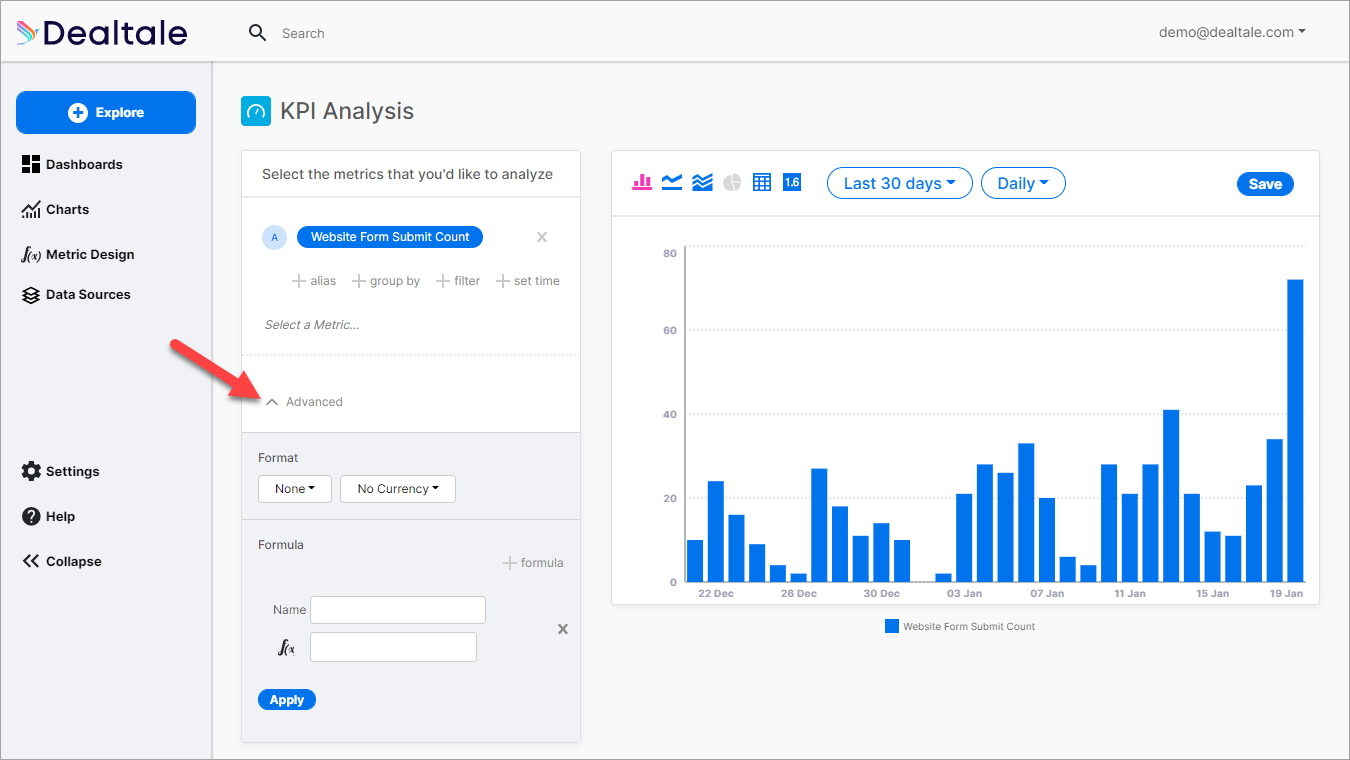# Format

You can format how to display your data on your chart according to number and currency formatting.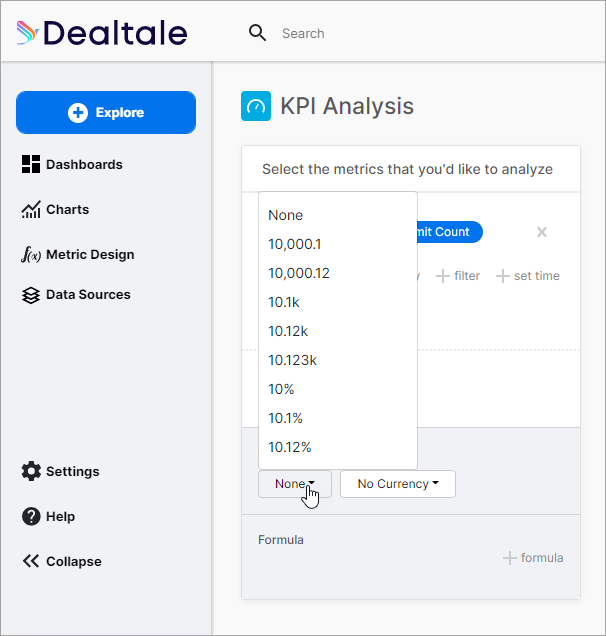Format

Description

10,000.1

Number

10,000.12

Number

10.1k

Number

10.12k

Number

10.123k

Number

10%

Percent

10.1%

Percent

10.12%

Percent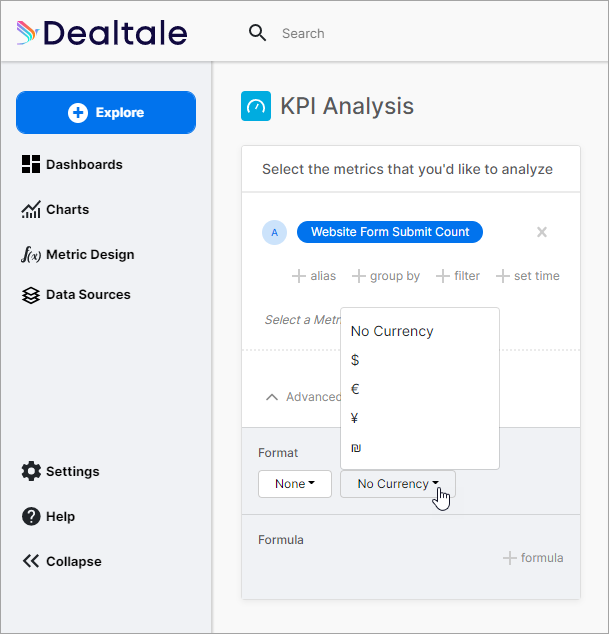Currency

Description

\$

United States Dollar

Euro

¥

Japanese Yen or Chinese Yuan

Israeli shekel

# Formula

You can use the Formula feature to calculate different Metrics and get specific results that are reflected on the graph on the right.

Note: You must select two or three metrics to be able to calculate a formula. Refer to Using KPIs for instructions.

1. Name your Formula in the field provided (1).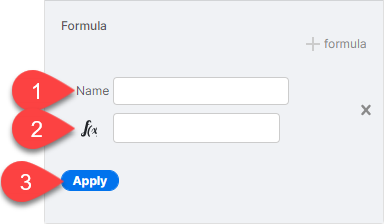1. Enter a formula in the f(x) field (2).
2. Click Apply (3).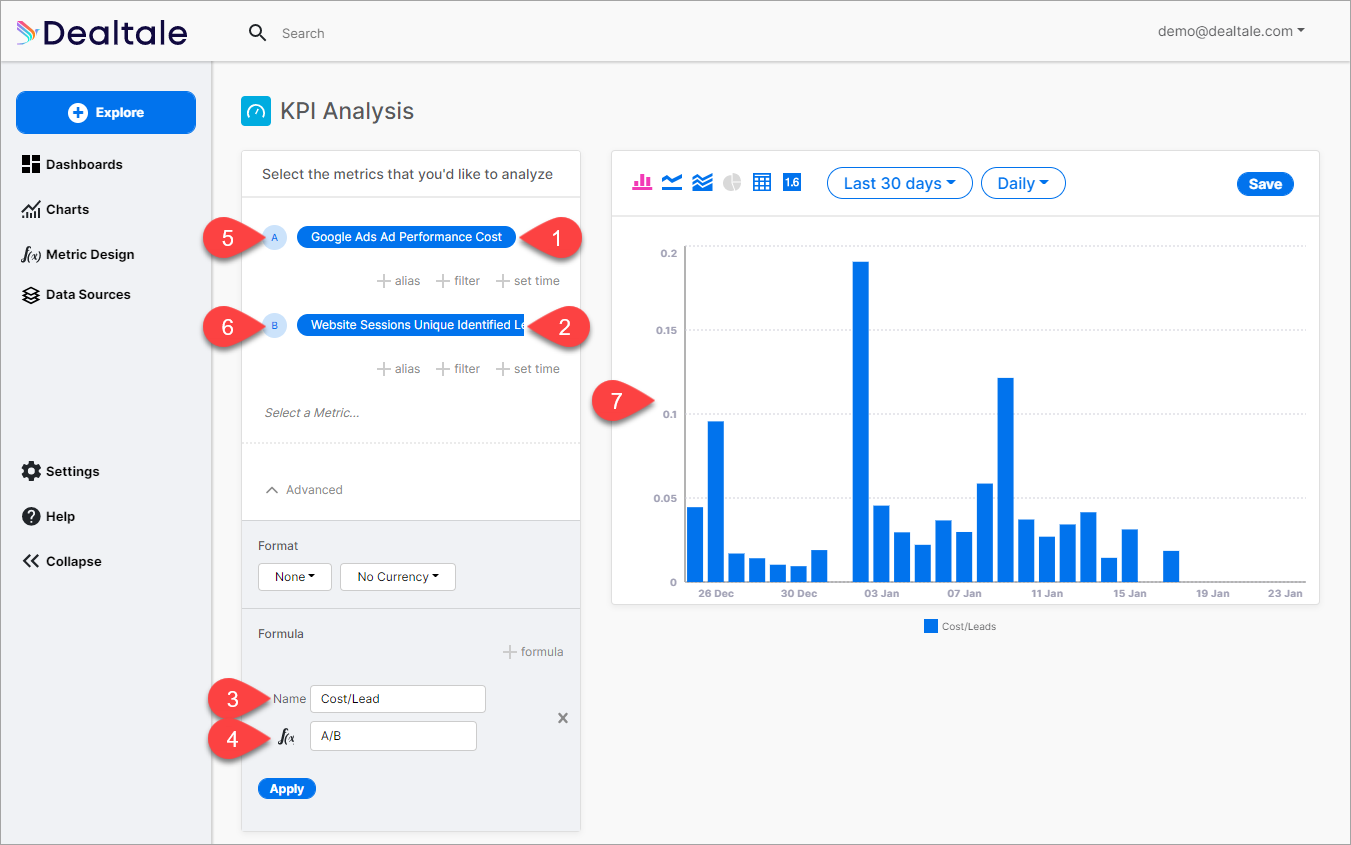In our example shown in the image above, we selected Cost in the first Metric (1) and Unique Identified Leads in the second Metric (2). We named the Formula "Cost/Lead."(3)

We now want to compute the cost per lead so we use the formula A divided by B (4):

`````` A/B

whereby:

A (5), the cost of Google Ads is divided by
B (6), the number of leads that came from Google Ads into your website.
``````

After clicking Apply, the result of the formula reflects on the graph on the right (7).